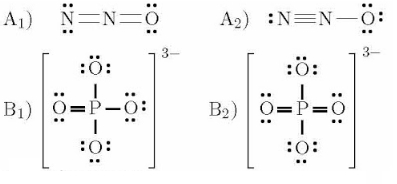# Problem: Consider the following pairs A and B of Lewis structures. Select the one from each pair that is likely to make the dominant contribution to a resonance hybrid.  1. A1 and B2 2. A2 and B1 3. A1 and B1 4. A2 and B2

###### FREE Expert Solution
90% (417 ratings)
###### Problem Details

Consider the following pairs A and B of Lewis structures. Select the one from each pair that is likely to make the dominant contribution to a resonance hybrid.

1. A1 and B2

2. A2 and B1

3. A1 and B1

4. A2 and B2What scientific concept do you need to know in order to solve this problem?

Our tutors have indicated that to solve this problem you will need to apply the Resonance Structures concept. You can view video lessons to learn Resonance Structures. Or if you need more Resonance Structures practice, you can also practice Resonance Structures practice problems.

What is the difficulty of this problem?

Our tutors rated the difficulty ofConsider the following pairs A and B of Lewis structures. Se...as medium difficulty.

How long does this problem take to solve?

Our expert Chemistry tutor, Dasha took 5 minutes and 4 seconds to solve this problem. You can follow their steps in the video explanation above.

What professor is this problem relevant for?

Based on our data, we think this problem is relevant for Professor Mandziuk & Orr's class at NYU.